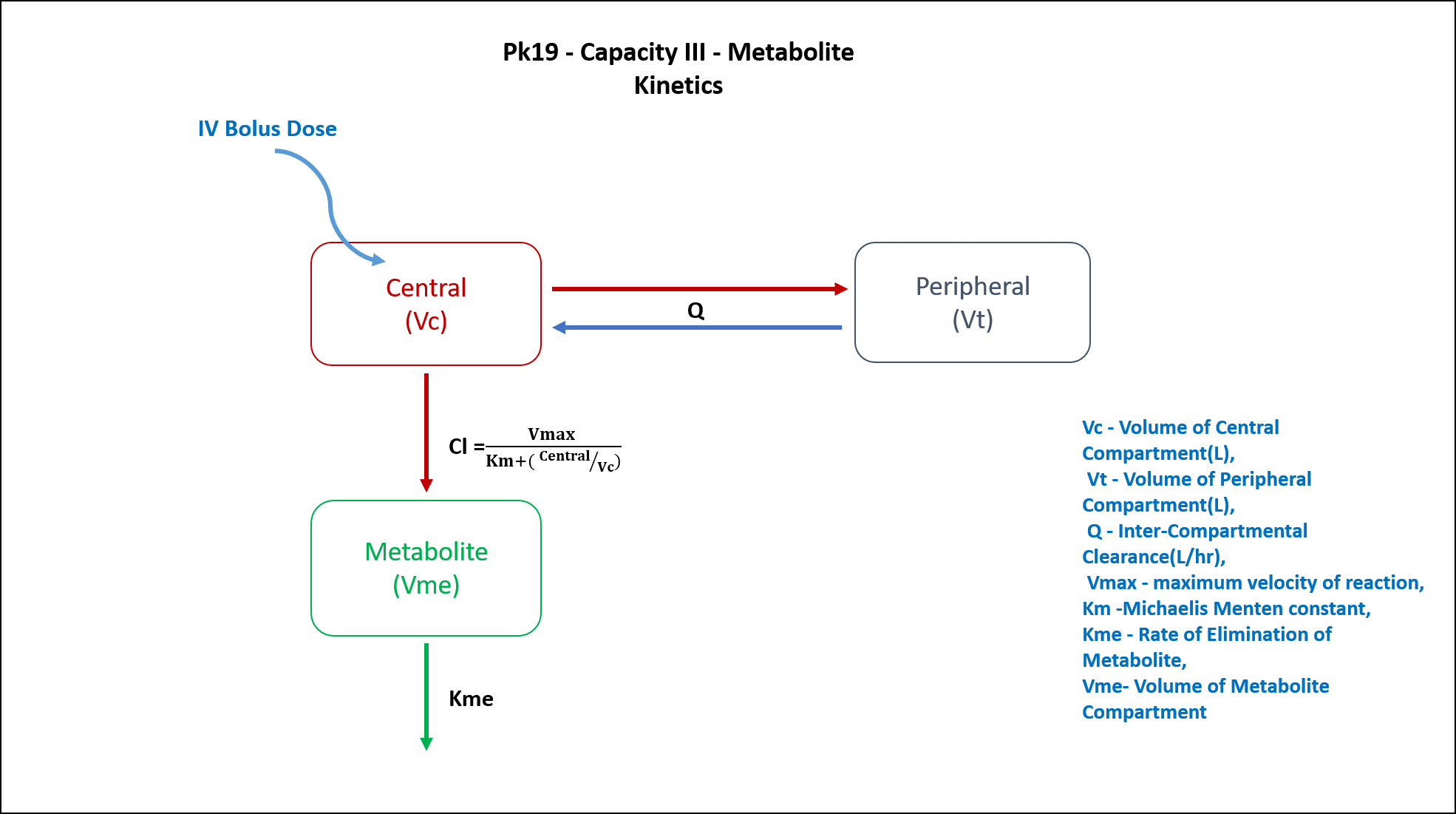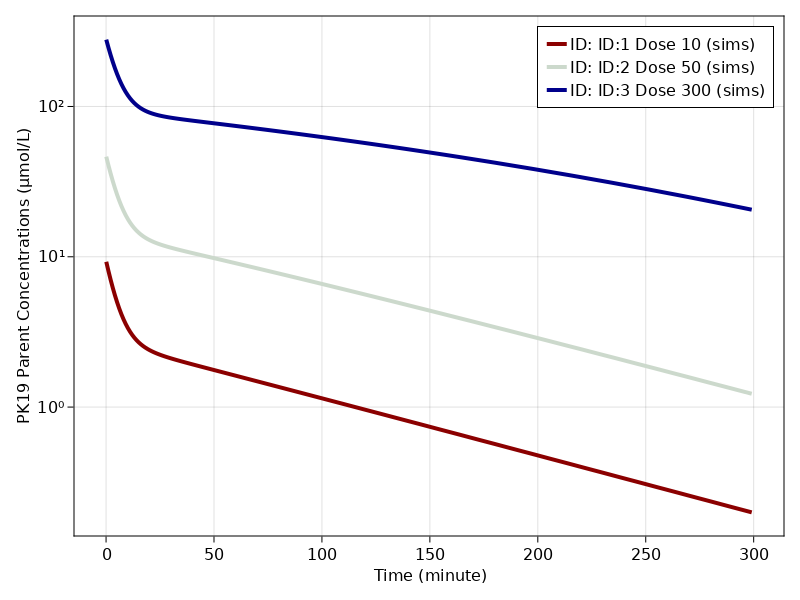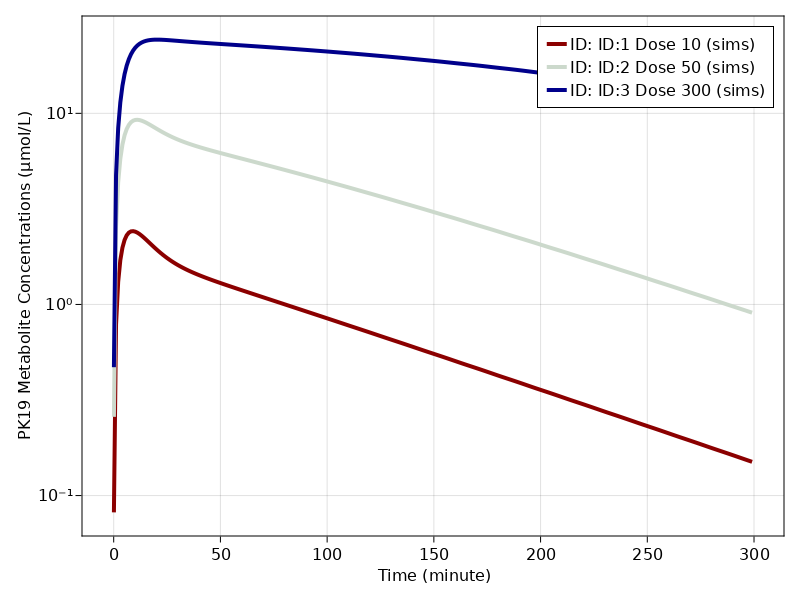# Exercise PK19 - Capacity III - Metabolite Kinetics

### Background

• Structural model - Two Compartment model with a Metabolite Compartment

• Route of administration - IV Bolus

• Dosage Regimen - 10μmol/kg, 50μmol/kg, 300μmol/kg

• Number of Subjects - 3### Learning Outcome

In this model, 3 different dose of the drug given as an IV Bolus to 3 different subjects, will help you estimate metabolite formation rate and elimination rate.

### Objectives

In this tutorial, you will learn how to build two compartment model, a drug undergoing capacity limited metabolite kinetics.

### Libraries

Call the "necessary" libraries to get start.

using Random
using Pumas
using PumasUtilities
using CairoMakie


### Model

In this two compartment model, we administer 3 different doses in 3 different subjects of a drug that undergoes metabolite kinetics.

pk_19           = @model begin
desc = "Non-linear formation of Metabolite Model"
timeu = u"hr"
end

@param begin
"Volume of Central Compartment (L/kg)"
tvvc        ∈ RealDomain(lower=0)
"Volume of Perpheral Compartment (L/kg)"
tvvp        ∈ RealDomain(lower=0)
"Inter-Compartmental Clearance (L/min)"
tvq         ∈ RealDomain(lower=0)
"Maximum Velocity of Reaction (μmol/min/kg)"
tvvmax      ∈ RealDomain(lower=0)
"Michaelis-Menten constant (μmol/L)"
tvkm        ∈ RealDomain(lower=0)
"Rate of Elimination of Metabolite (min⁻¹)"
tvkme       ∈ RealDomain(lower=0)
"Volume of Metabolite Compartment (L/kg)"
tvvme       ∈ RealDomain(lower=0)
Ω           ∈ PDiagDomain(7)
"Proportional RUV - Plasma"
σ_prop_cp  ∈ RealDomain(lower=0)
"Proportional RUV - Metabolite"
σ_prop_met ∈ RealDomain(lower=0)
end

@random begin
η           ~ MvNormal(Ω)
end

@pre begin
Vc          = tvvc * exp(η)
Vp          = tvvp * exp(η)
Q           = tvq * exp(η)
Vmax        = tvvmax * exp(η)
Km          = tvkm * exp(η)
Kme         = tvkme * exp(η)
Vme         = tvvme * exp(η)
end

@vars begin
VMKM       := Vmax/(Km+(Central/Vc))
end

@dynamics begin
Central'    = -VMKM*(Central/Vc) - (Q/Vc)*Central + (Q/Vp)*Peripheral
Peripheral' = (Q/Vc)*Central - (Q/Vp)*Peripheral
Metabolite' = VMKM*(Central/Vc) - Kme*Metabolite
end

@derived begin
cp          = @. Central/Vc
"""
Observed Plasma Concentration (μmol/L)
"""
dv_cp       ~ @. Normal(cp, cp*σ_prop_cp)
met         = @. Metabolite/Vme
"""
Observed Metabolite Concentration (μmol/L)
"""
dv_met      ~ @. Normal(met, met*σ_prop_met)
end
end

PumasModel
Parameters: tvvc, tvvp, tvq, tvvmax, tvkm, tvkme, tvvme, Ω, σ_prop_cp, σ_
prop_met
Random effects: η
Covariates:
Dynamical variables: Central, Peripheral, Metabolite
Derived: cp, dv_cp, met, dv_met
Observed: cp, dv_cp, met, dv_met


### Parameters

The parameters are as given below. tv represents the typical value for parameters.

• $Vc$ - Volume of Central Compartment (L/kg)

• $Vp$ - Volume of Peripheral Compartment (L/kg)

• $Q$ - Inter-Compartmental Clearance (L/min)

• $Vmax$ - Maximum Velocity of Reaction (μmol/min/kg)

• $Km$ - Michaelis-Menten constant (μmol/L)

• $Kme$ - Rate of Elimination of Metabolite (min⁻¹)

• $Vme$ - Volume of Metabolite Compartment (L/kg)

• $Ω$ - Between Subject Variability

• $σ$ - Residual error

param = ( tvvc        = 1.06405,
tvvp        = 2.00748,
tvq         = 0.128792,
tvvmax      = 1.64429,
tvkm        = 54.794,
tvkme       = 0.145159,
tvvme       = 0.290811,
Ω           = Diagonal([0.0,0.0,0.0,0.0,0.0,0.0,0.0]),
σ_prop_cp  = 0.12,
σ_prop_met = 0.12)

(tvvc = 1.06405, tvvp = 2.00748, tvq = 0.128792, tvvmax = 1.64429, tvkm = 5
4.794, tvkme = 0.145159, tvvme = 0.290811, Ω = [0.0 0.0 … 0.0 0.0; 0.0 0.0
… 0.0 0.0; … ; 0.0 0.0 … 0.0 0.0; 0.0 0.0 … 0.0 0.0], σ_prop_cp = 0.12, σ_p
rop_met = 0.12)


### Dosage Regimen

Three Subjects were adminitered with three different doses of 10μmol/kg, 50μmol/kg and 300μmol/kg.

dose  = [10, 50, 300]
ids   = ["ID:1 Dose 10", "ID:2 Dose 50", "ID:3 Dose 300"]
ev(x) = DosageRegimen(dose[x], cmt = 1, time = 0)

pop = map(zip(1:3, ids)) do (i, id)
return Subject(id = id, events = ev(i), observations = (cp = nothing, met = nothing))
end

Population
Subjects: 3
Observations: cp, met


### Simulation

We will simulate the parent plasma concentration and metabolite plasma concentration.

Random.seed!(123)
sim_pop3_sub = simobs(pk_19, pop, param, obstimes = 0.1:1:300)


### Visualization

fig = Figure()
ax, p1 = sim_plot(fig[1,1], pk_19,
sim_pop3_sub,
observations = :cp,
color = :redsblues,
linewidth = 4,
axis = (xlabel = "Time (minute)",
ylabel = "PK19 Parent Concentrations (μmol/L)",
xticks = 0:50:300, yscale = log10))

axislegend(ax)
figfig = Figure()
ax, p1 = sim_plot(fig[1,1], pk_19,
sim_pop3_sub,
observations = :met,
color = :redsblues,
linewidth = 4,
axis = (xlabel = "Time (minute)",
ylabel = "PK19 Metabolite Concentrations (μmol/L)",
xticks = 0:50:300, yscale = log10))

axislegend(ax)
fig